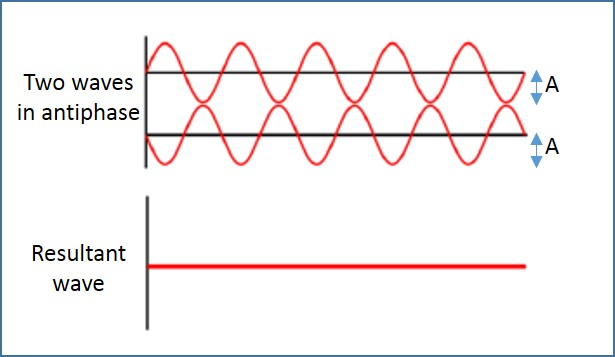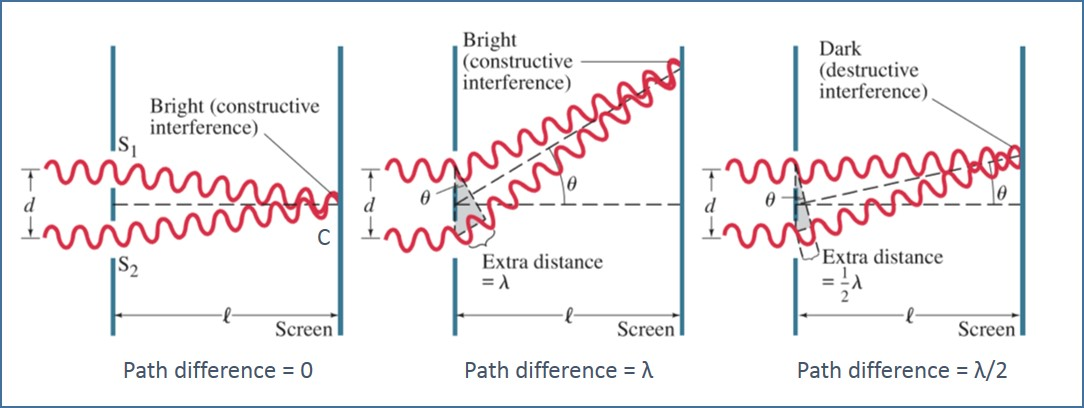# Superposition Of Waves

## Introduction

If two or more waves collide, they are said to interfere. Interference is a property of waves. In this article, properties of the resultant waves created in interference is explained.

A. Superposition of waves

The principle of superposition states that when two or more waves meet at a point, the resultant displacement at that point is equal to the sum of the displacements of the individual waves at that point.

Consider two waves that arrive in phase as shown in Figure 1. Their crests arrive at exactly the same time. Hence, they interfere constructively. A resultant wave is produced, which has a crest much larger than the two individual waves, and the troughs are deeper. If the two incoming waves that are in phase have amplitude of A, then the resultant wave has an amplitude of 2A. The frequency of the resultant is the same as that of the incoming waves.Figure 1: Constructive Interference

Consider two waves that arrive in antiphase (with a phase difference of π or 180°) as illustrated in Figure 2. The crest of one wave and the trough of another wave arrive at exactly the same time. Hence, they interfere destructively. A resultant wave is produced, which has a smaller amplitude. If the two incoming waves that are in antiphase have amplitude of A, then the resultant wave has an amplitude of zero. The frequency of the resultant is the same as that of the incoming waves.Figure 2: Destructive Interference

It can be noted that as the displacement is a vector quantity, the direction of the wave must be noted when the individual waves are added. Stationary waves are formed by two waves with the same frequency travelling in opposite directions.

Figure 3 shows the interference of waves from two point sources, S1 and S2. The point C is equidistant from S1 and S2 and hence, the path difference is 0. If the waves start in phase at S1 and S2, they will arrive at C combining constructively, producing maximum disturbance at C.

Points D and E are at different distances from S1 and S2 and hence there is a path difference between the waves. If the path difference is a whole number of wavelengths (1λ, 2λ, 3λ, and so on), the waves are in phase and combine constructively. A resultant with maximum displacement is formed. If the path difference is an odd number of half-wavelengths (1λ/2, 3λ/2, 5λ/2, and so on), the waves are in antiphase and combine destructively. A resultant with minimum displacement is formed. The maximum and minimum displacements are called fringes. This collection of fringes produced by superposition of overlapping waves is called an interference pattern.Figure 3: Path Difference

B. Summary

• The principle of superposition states that when two or more waves meet at a point, the resultant displacement at that point is equal to the sum of the displacements of the individual waves at that point.
• If the two incoming waves that are in phase have amplitude of A, then the resultant wave has an amplitude of 2A.
• If the two incoming waves that are in antiphase have amplitude of A, then the resultant wave has an amplitude of zero.
• Stationary waves are formed by two waves with the same frequency travelling in opposite directions.